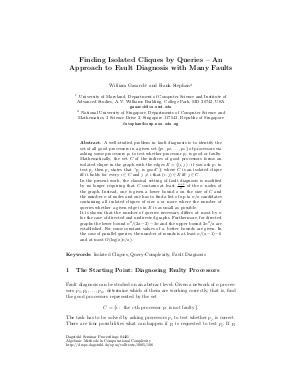Document# Finding Isolated Cliques by Queries – An Approach to Fault Diagnosis with Many Faults

### Authors William Gasarch, Frank Stephan## File

DagSemProc.04421.3.pdf
• Filesize: 236 kB
• 16 pages

## Cite As

William Gasarch and Frank Stephan. Finding Isolated Cliques by Queries – An Approach to Fault Diagnosis with Many Faults. In Algebraic Methods in Computational Complexity. Dagstuhl Seminar Proceedings, Volume 4421, pp. 1-16, Schloss Dagstuhl - Leibniz-Zentrum für Informatik (2005)
https://doi.org/10.4230/DagSemProc.04421.3

## Abstract

A well-studied problem in fault diagnosis is to identify the set of all good processors in a given set $\{p_1,p_2,\ldots,p_n\}$ of processors via asking some processors $p_i$ to test whether processor $p_j$ is good or faulty. Mathematically, the set $C$ of the indices of good processors forms an isolated clique in the graph with the edges $E = \{(i,j):$ if you ask $p_i$ to test $p_j$ then $p_i$ states that $p_j$ is good''$\}$; where $C$ is an isolated clique iff it holds for every $i \in C$ and $j \neq i$ that $(i,j) \in E$ iff $j \in C$. In the present work, the classical setting of fault diagnosis is modified by no longer requiring that $C$ contains at least $\frac{n+1}{2}$ of the $n$ nodes of the graph. Instead, one is given a lower bound $a$ on the size of $C$ and the number $n$ of nodes and one has to find a list of up to $n/a$ candidates containing all isolated cliques of size $a$ or more where the number of queries whether a given edge is in $E$ is as small as possible. It is shown that the number of queries necessary differs at most by $n$ for the case of directed and undirected graphs. Furthermore, for directed graphs the lower bound $n^2/(2a-2)-3n$ and the upper bound $2n^2/a$ are established. For some constant values of $a$, better bounds are given. In the case of parallel queries, the number of rounds is at least $n/(a-1)-6$ and at most $O(\log(a)n/a)$.
##### Keywords
• Isolated Cliques
• Query-Complexity
• Fault Diagnosis

## Metrics

• Access Statistics
• Total Accesses (updated on a weekly basis)
0